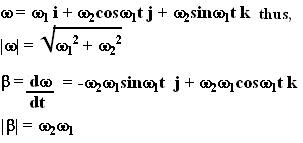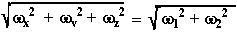## Saturday, August 4, 2007

### Irodov Problem 1.55

We shall solve this problem in two ways, i) using our basic knowledge and ii) using Euler's angles (for details see here). The reason is more general problems in rotation can be solved using Euler's angles better.

Method 1: Without using Euler's angles:Let us arbitrarily assume that body B1 rotates with an angular velocityabout the z-axis and the other body B2 rotates with an angular velocityabout the x-axis. To the person on B1 he himself is stationary and everything else in the universe around him is rotating atin the opposite direction about the x-axis. So to the person on B1, i) the axis of body B2 rotates about the x-axis with angular velocityand in addition ii) is spinning about its own axis with the angular velocity vector. In other words, the angular velocity. Basically, as seen from one of the rotating bodies, the second body undergoes simultaneous rotation along two perpendicular axis. This is indicated in the figure. The net result is that relative to one body the other seems to undergo precession at a constant rate.

The net angular velocity of B2 as seen from B1 and hence acceleration are thus given by,Method 2: Using Euler's angles:
This problem is best solved with the knowledge of Euler's angles In terms of Euler's angles we have,In Euler angles,is rotation about the z-axis andis rotation about the x-axis. Then,Thus, now, we can write the angular velocity vector of one body relative to the other as,The magnitude of this angular velocity vector is the Euclidean norm of the vector and is given by,What this means is that the magnitude of the velocity vector itself never changes, only its direction changes. This is an nice example of precession. The rotation of the angular velocity vector then induces an angular acceleration vector.

The angular acceleration vector can be obtained by differentiating the angular velocity vector with respect to time and is given by,Now we can compute the net angular acceleration using the Euclidean norm of the acceleration vector as,#### 1 comment:Anonymous said...

I loved method 1!. It was very helpful. I have been trying to understand this for ages now, and I finally did. Method 2 is tricky to understand though...